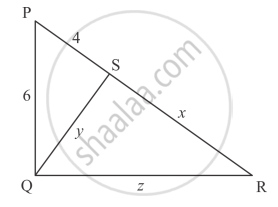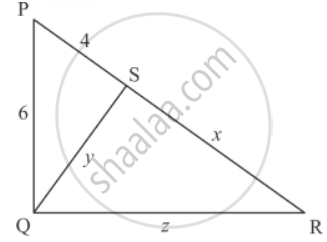Advertisement Remove all ads

# In Each of the Figures Given Below, an Altitude is Drawn to the Hypotenuse by a Right-angled Triangle. the Length of Different Line-segment Are Marked in Each Figure. Determine X, Y, Z in Each Case. - Mathematics

Sum

In each of the figures given below, an altitude is drawn to the hypotenuse by a right-angled triangle. The length of different line-segment are marked in each figure. Determine x, y, z in each case.Advertisement Remove all ads

#### Solution

Δ PQR  is a right triangle, right angled at Q6+z^2 = (4+x)^2

6+z^2=16+x^2+8x

z^2-x^2-8x=16-36

z^2-x^2-8x=16-36

z^2-x^2-8x=-20......(1)

Δ QSP  is a right triangle right angled at S

QS^2+PS^2=PQ^2

y^2+4^2=6^2

y^2+16=36

y^2=36-16

y^2=20

y=sqrt20

y=sqrt(2xx2xx5)

y=2sqrt5

Δ QSR  is a right triangle right angled at S

QS^2+RS^2=QR^2

y^2+x^2=z^2..........(2)

Now substituting  y^2+x^2=z^2 in equation (i) we get

y^2+x^2-x^2-8x=-20

20-8x=-20

-8x=-20-20

-8x=-40

x=40/8

x=5

Now substituting  x = 5 and  y^2=20 in equation (ii) we get

y^2+x^2=z^2

20+5^2=z^2

20+25=z^2

45=z^2

sqrt(3xx3xx5)=z^2

3sqrt5=z

Hence the value of x, y and z are  5,2sqrt5,3sqrt5

Concept: Triangles Examples and Solutions
Is there an error in this question or solution?
Advertisement Remove all ads

#### APPEARS IN

RD Sharma Class 10 Maths
Chapter 7 Triangles
Q 21.2 | Page 126
Advertisement Remove all ads

#### Video TutorialsVIEW ALL 

Advertisement Remove all ads
Share
Notifications

View all notifications

Forgot password?
Course